Sie sind hier: ICP » R. Hilfer » Publikationen

VI Model Calculations

[68.1.2.1] This section returns to eq. (3.2) and presents numerical solutions. [68.1.2.2] This is intended as a case study exploring the relationship between the statistics of local geometries and bulk dielectric behavior. [68.1.2.3] The main focus will be on dielectric enhancement. [68.1.2.4] To solve eq. (3.2) for εω, one must know the geometric input functions μϕ, λϕ and local dielectric responses εCω;ϕ, εBω;ϕ. [68.1.2.5] Unfortunately, no experimental data are available at present, and geometric modeling has to be used instead.

A Local dielectric response

[68.1.3.1] The hypothesis of local simplicity states that the local geometries are simple and that the effective local dielectric constants are insensitive to geometrical details other than local porosity. [68.1.3.2] The simplest isotropic local geometry is spherical. [68.2.0.1] For conducting local geometries, a water-coated spherical rock grain will serve as the local model. [68.2.0.2] For blocking geometries a rock-coated spherical water pore is employed. [68.2.0.3] In the notation of Sec. IV, this means

 εC⁢u;ϕ =εW⁢1-1-ϕ1-εR/εW-1-13⁢ϕ, (6.1) εB⁢u;ϕ =εR⁢1-ϕ1-εW/εR-1-13⁢1-ϕ. (6.2)

[68.2.0.4] In the low-frequency limit, one obtains for the conducting geometry

 σC′⁢0;ϕ=σW′⁢2⁢ϕ3-ϕ=23⁢σW′⁢ϕ⁢1+13⁢ϕ+…, (6.3)

thereby identifying C1 in eq. (4.8) as C1=23σW. [68.2.0.5] The real dielectric constant is found as

 εC′⁢0;ϕ=εW′-1-ϕ1-13⁢ϕ2⁢εW′⁢1-13⁢ϕ-εR′. (6.4)

[68.2.0.6] For the blocking geometry, the dc limit gives σB0;ϕ=0, in agreement with eq. (4.6), and

 εB′⁢0;ϕ=εR′⁢1+2⁢ϕ1-ϕ, (6.5)

for ϕ<1, identifying B1=1/εR in eq. (4.9), and εB0;ϕ=εW, for ϕ=1. [68.2.0.7] Note the presence of the thin-plate divergence in the ϕ1 limit.

B Local porosity distribution

[68.2.1.1] It was mentioned repeatedly that no experimental data for μϕ are available to the author at present. [68.2.1.2] A qualitative guideline for porous media resulting from spinodal decomposition might be the shape of the order-parameter distribution calculated in Ref.  which suggests in particular that μϕ can be bimodal.

[68.2.2.1] For the subsequent calculations, a simple mixture of two β distributions has been used. [68.2.2.2] The analytic expression reads

 μ⁢ϕ=ω⁢Γ⁢μ1+ν1Γ⁢μ1⁢Γ⁢ν1⁢1-ϕμ1-1⁢ϕν1-1+1-ω⁢Γ⁢μ2+ν2Γ⁢μ2⁢Γ⁢ν2⁢1-ϕμ2-1⁢ϕν2-1, (6.6)

where 0ω1, μ>0, ν>0 and Γx denotes Euler’s Γ function. [68.2.2.3] The bulk porosity is then given as

 ϕ¯=ω⁢ν1μ1+ν1+1-ω⁢ν2μ2+ν2. (6.7)

[68.2.2.4] For μ,ν>1, the β densities are bell shaped, and for μ,ν>1 they diverge at the limits.

[68.2.3.1] Eight different local porosity distributions are compared in the calculations. [68.2.3.2] All of them are chosen such that they give the same bulk porosity ϕ¯=0.1. [68.2.3.3] The values of the parameters are listed in Table 1, and the densities [page 69, §0]    themselves are displayed graphically in fig. 1. [69.1.0.1] Each distribution is identified by a number and a line style as indicated in the inset of Fig. 1. ϕ¯i (i=1,2) are the partial porosities νi/νi+μi in eq. (6.7). [69.1.0.2] The uniform distribution carries number 1 and is identified by a thin dot-dashed line. [69.1.0.3] Number 2 represents the strongly peaked case and is identified by a wide dashed line, and so on.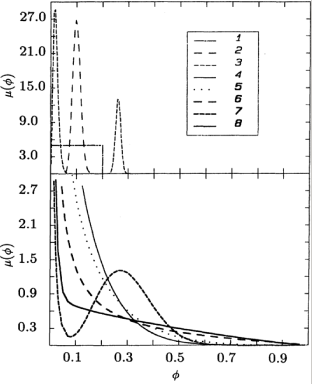Figure 1: Eight porosity densities with parameters given in Table 1.
Table 1: Paramenter values for the eight different forms of μϕ displayed in Fig. 1 and used in the model calculations. The parameters ω, μ1, ν1, μ2 and ν2 are those of Eq. (6.6). ϕ¯1, ϕ¯2, var1 and var2 are the average and variance for μ1, μ2, if μ in Eq. (6.6) is written as μ=ωμ1+1-ωμ2. The rows labeled ϕ¯, variance, and skewness contain the mean, variance and skewness for the eight forms of μϕ.
Curve No. 1 2 3 4 5 6 7 8
ω 1 1 2/3 1 1 1 2/3 2/3
μ1 1.0 360.0 191.1 7.2 4.5 1.8 28.8 58.6
ν1 1.000 40.000 3.900 0.800 0.500 0.200 0.087 0.176
μ2 1423.0 13.9 2.24
ν2 500.00 6.00 0.96
ϕ¯1 0.1 0.1 0.02 0.1 0.1 0.1 0.003 0.003
ϕ¯2 0.26 0.294 0.294
var1 0.00333 0.00024 0.00010 0.00010 0.01500 0.03000 0.00010 0.00005
var2 0.00010 0.01000 0.05000
ϕ¯ 0.1 0.1 0.1 0.1 0.1 0.1 0.1 0.1
Variance 0.00333 0.00024 0.01290 0.01000 0.01500 0.03000 0.02213 0.03541
Skewness 0 0.2657 0.6993 1.6004 1.8679 2.3124 1.1588 2.1176

[69.1.1.1] The choices presented in Fig. 1 are arbitrary. [69.1.1.2] The reader should bear in mind, however, that μϕ is easily measureable and cannot be adjusted to fit experimentally observed dielectric data when comparing the theory with experiment. [69.1.1.3] The choices for μϕ presented here are intended as a case study illustrating different possibilities that might occur in real or artificial experimental systems. [69.2.0.1] The highly peaked μϕ (curve 2) represents the limit of weak disorder. [69.2.0.2] Remember that μϕ=δϕ-ϕ¯ for ordered systems. [69.2.0.3] Curve 2 gives a reference to which other distributions can be compared. [69.2.0.4] The distributions 4, 5 and 6 have been chosen divergent at ϕ=0 with exponents 0.8, 0.5 and 0.2 as examples for distributions whose inverse first moment does not exist. [69.2.0.5] Curves 3, 7 and 8 demonstrate the fact that μϕ itself might be “of percolation type.” [69.2.0.6] This occurs if the porous medium contains two types of porosity or regions of very different porosities. [69.2.0.7] For curve 3 the ratio between the two porosities is roughly 100, and the inverse first moment of μϕ exists. [69.2.0.8] For curves 7 and 8, the ratio roughly 1000, and the densities diverge at ϕ=0. [69.2.0.9] In all cases the distributions were chosen critical in the sense that the weight for the higher-porosity component is 13.

C Local percolation probability

[69.2.1.1] The local percolation probabilities λϕ can be measured simultaneously with the local porosity distribution μϕ. [69.2.1.2] However, such a measurement is more difficult because it requires the approximate reconstruction of the three-dimensional pore space from parallel two-dimensional sections. [69.2.1.3] For this reason geometric modeling of porous media is most important for this quantity.

[69.2.2.1] Three simple models will be compared in the calculations: the uniformly connected model (UCM), the central pore model (CPM), and the grain consolidation model (GCM).

1 Uniformly connected models

[69.2.3.1] In these models λϕ equals a constant, i.e.,

 α⁢ϕ=p. (6.8)

[69.2.3.2] In the simplest case, the fully connected model, p=1. [69.2.3.3] This means that all local geometries are assumed to be [page 70, §0]    conducting. [70.1.0.1] In addition, the case p=12 will also be investigated.

2 Central pore model

[70.1.1.1] Consider a cubic cell of volume 1 filled with rock. [70.1.1.2] Inside the cubic cell a centered cubic pore of side length a (0a1) is cut out. [70.1.1.3] Now a random process is used to drill cylindrical pores with square cross section from the faces of the cube toward the central pore. [70.1.1.4] Sometimes these pores will connect to the central pore, and sometimes not. [70.1.1.5] The random process starts with the choice of an arbitrary face of the cube. [70.1.1.6] Now choose a random number r between 0 and 12. [70.1.1.7] If r>121-a, a pore with square cross section of side length b (0ba) is drilled from the center of the face all the way to the central pore. [70.1.1.8] The central pore had volume a3, and the connection pore has the volume 12b21-a. [70.1.1.9] If the random number fulfills f<121-a, then the face is not pierced, but instead the same volume 12b21-a is removed from the wall in such a way that the resulting pore space remains disconnected from the pore space connected to the central pore. [70.1.1.10] This process is repeated for all six faces of the cube. [70.1.1.11] The cubic symmetry is not essential, and a model with different symmetry can be defined similarly.

[70.1.2.1] The result of the process described above is a cubic cell whose porosity can be expressed in terms of the side length a of the central pore and the ratio R=b/a as

 ϕ=a3+3⁢b2⁢1-a=a3⁢1-3⁢R2+3⁢R2⁢a2. (6.9)

[70.1.2.2] According to the definitions in Section II, the cell is called percolating if there exist at least one path within the pore space connecting a face to a face different than itself. [70.1.2.3] To obtain λϕ the probability that either no or exactly one face is pierced has to be calculated. [70.1.2.4] This probability euqals 1-λϕ. [70.1.2.5] Clearly,

 1-λ=1-a6+a⁢1-a5=1-a5. (6.10)

[70.1.2.6] Thus, in the central pore model,

 λ⁢ϕ,R=1-1-a⁢ϕ,R5, (6.11)

where aϕ,R is that root of eq. (6.9), which fulfills 0a1 for all 0ϕ1 and 0R1. [70.1.2.7] For R=1, i.e., b=a, it follows that ϕa2 and thus aϕ1/2, resulting in λϕϕ1/2 for small ϕ. [70.1.2.8] On the other hand, for R0 one finds aϕ1/3 for ϕ0 and thus λϕϕ1/3 for ϕ0. [70.1.2.9] Thus the general conclusion for the central pore model is that

 λ⁢ϕ∝ϕγ, (6.12)

where γ can range between 12 and 13.

3 Grain consolidation model

[70.1.3.1] The grain consolidation model was proposed as a simple geometrical model for diagenesis.[70.1.3.2] Its main observation is the existence and smallness of the percolation threshold in regular and random bead packings when the bead radii are increased. [70.1.3.3] In fact, the model has recently been modified such that the critical porosity at which conduction ceases can be arbitrarily small.[70.2.0.1] For regular bead packings, this implies

 λ⁢ϕ=0for⁢ϕ<ϕc1for⁢ϕ>ϕc. (6.13)

[70.2.0.2] For random packings λϕ will be smoothed out around ϕc. [70.2.0.3] For simplicity, in this paper eq. (6.13) will be used with uphic=0.05.

[70.2.1.1] The most important aspect of λϕ is that it determines the control parameter p. [70.2.1.2] According to eq. (6.12), its behavior near ϕ=0 can influence the exponent α in (5.10c). [70.2.1.3] Note that for the grain consolidation model the form of λϕ always implies that condition (5.10a) is fulfilled, and universal behavior is expected. [70.2.1.4] The half-connected model in the uniformly connected model class is included to demonstrate the influence of the thin-plate effect. [70.2.1.5] The shape of λϕ in all other cases gives extremely small probability to blocking geometries with high porosities. [70.2.1.6] This is expected to be generally true for interparticle porosity. [70.2.1.7] This is expected to be generally true for interparticle porosity. [70.2.1.8] However, the secondary pore space in real rocks may contain a significant fraction of high-porosity blocking geometries.

D Numerical results

[70.2.2.1] Numerical solutions to eq. (3.2) were obtained using an iterative technique. [70.2.2.2] The iteration was stopped whenever εnω-εn-1ω<10-7. [70.2.2.3] In Figs. 2-5 selected results for εω are presented. [70.2.2.4] Figure 2 presents the uniformly connected model with p=1, Figure 3 the uniformly connected model with p=12. [70.2.2.5] Figure 4 gives the results for the central pore model with R=0.922, and Fig. 5 those for the grain consolidation model with ϕc=0.05. [70.2.2.6] In each figure the line styles correspond to the line styles of the local porosity distributions displayed in Figure 1. [70.2.2.7] Parts (a) of each figure shows log10εω as a function of log10ω in the upper graph and log10σω in the lower graph. [70.2.2.8] In addition, the inset in the upper right-hand corner displays the local percolation probability λϕ for 0ϕ12. [70.2.2.9] The vertical scale for the inset is always 0λ2. [70.2.2.10] Part (b) display in the upper graph -dlog10εω/dlog10ω versus log10ω and dlog10σω/dlog10ω in the lower plot. [70.2.2.11] These quantities give a more sensitive representation of the dispersion and show at the same time the values of “local exponents”. [70.2.2.12] In all figures frequency is measured in units of the relaxation frequency of water as discussed in Section IV. [70.2.2.13] All plots are given over ten frequency decades with a resolution of five points per decade.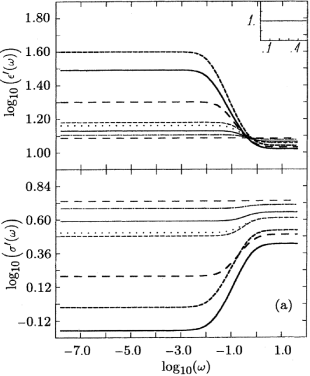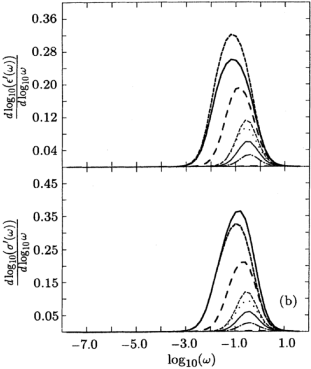Figure 2: (a) Real part of the complex dielectric constant (upper graph) and real part of complex conductivity (lower graph) vs frequency in a doubly logarithmic plot. Each graph contains eight curves whose line style corresponds to the local porosity densities displayed in Figure 1. The inset shows the local percolation probability λϕ used in the calculation, in this case that of the uniformly conducting model with p=1. The vertical axis of the inset ranges form λ=0 to 2, the horizontal axis from ϕ=0 to 0.5. (b) Derivativs of the curves in (a).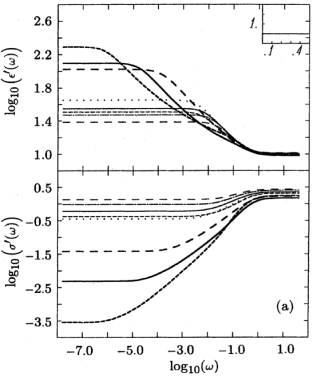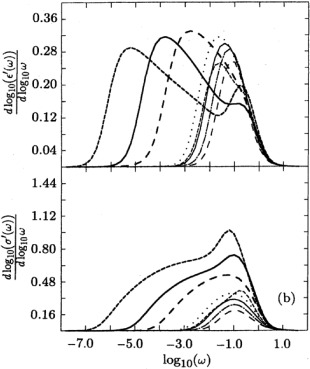Figure 3: Same as Figure 2 for the uniformly conducting model with p=12.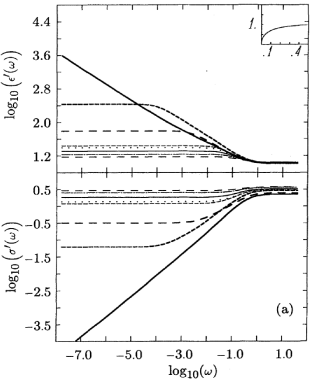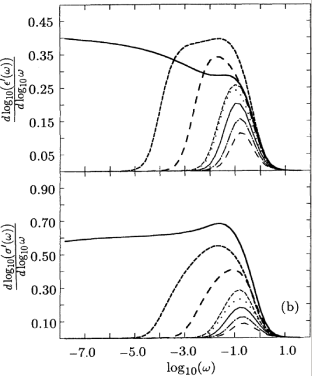Figure 4: Same as Figure 2 for the central pore model with R=0.922.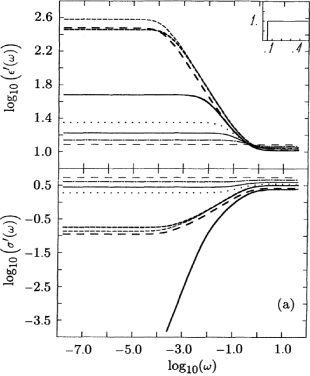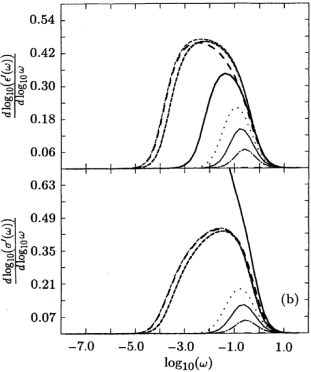Figure 5: Same as Figure 2 for the grain consolidation model with ϕc=0.05.

E Discussion

[70.2.3.1] It is obvious from Figs. 2-5 [especially part (b)] that the low-frequency dielectric response depends sensitively on the details of μϕ and λϕ. [70.2.3.2] A general discussion is difficult, because the response is always a mixture between three basic mechanisms each of which can give significant dielectric dispersion. [page 71, §0]    [71.1.0.1] The first mechanism is the dispersion resulting from the disorder in μϕ itself. [71.1.0.2] The second mechanism is the despersion resulting from p, i.e., from percolation geometry. [71.1.0.3] The third mechanism is the dispersion resulting from the behavior of λϕ in the ϕ1 limit, i.e., the thin-plate effect.

[71.1.1.1] The absolute dispersion for all figures is collected in Table 2. [71.1.1.2] Δε is defined as Δε=ε0-ε, while Δσ=σ-σ0.

[71.2.1.1] Before discussing the three mechanisms, it is important to note that the bulk porosity ϕ¯ does not influence the shape of the response curves if it is changed without changing the shape of μϕ. [71.2.1.2] Instead, it determines an overall frequency shift for the frequency region over which the dispersion occurs. [71.2.1.3] As ϕ¯ is lowered, this region is shifted toward lower frequencies. [71.2.1.4] This observation together with the fact that all μϕ give the same bulk porosity of 0.1 shows that the bulk porosity by itself cannot be used to characterize the dielectric response. [71.2.1.5] In particular, there is no theoretical basis for Archie’s law [eq. (5.27)] if interpreted as a relation between dc conductivity and bulk porosity (see Section V.D for a discussion).

[page 72, §0]

[page 73, §0]    [73.1.1.1] A second observation is that in all figures the high-frequency real dielectric constant ε is not very sensitive to the details of μϕ. [73.1.1.2] This is a consequence of the fact that for low ϕ¯ the local dielectric constants εB and εC must both approach εR.

[73.1.2.1] The first mechanism, dispersion from the form of μϕ, can be studied in pure form when λϕ=1, and the corresponding results are shown in Figure 2. [73.1.2.2] In this case there are no blocking local geometries; i.e., p=1 according to eq. (5.7). [73.1.2.3] If μϕ is highly peaked as in curve 2, then the system is only weakly disordered, and there is almost no visible disperion with the amount of disorder contained in μϕ. [73.1.2.4] In fact, distributions with power-law divergences at ϕ0 or with percolation structure generate the strongest dispersion, as can be seen from curves 3 and 5-8 in figure 2. [73.1.2.5] Table 2 shows that the dispersion varies almost three orders of magnitude between the different distributions. [73.1.2.6] Note that relatively similar local porosity distributions such as curves 6 and 8 can have very different dielectric response. [73.1.2.7] On the other hand, very different shapes for μϕ can give similar εω, as demonstrated by curves 3 and 5. [73.1.2.8] This shows that the dielectric response by itself does not contain a full geometric characterization of the pore space, and it needs always to be complemented with additional physical or geometrical information. [73.1.2.9] This is not too surprising. [73.1.2.10] Indeed, it is more surprising that when the dielectric response becomes large it is also very sensitive to geometric details. [73.1.2.11] This is the case for dielectric enhancement near the percolation threshold or as a result of the thin-plate effect.

[73.1.3.1] Consider the thin-plate mechanism. [73.1.3.2] It requires the presence of blocking geometries of high porosity. [73.1.3.3] Mathematically, this means λϕ1 for large ϕ. [73.1.3.4] As a simple illustration, Figure 3 displays the results for the uniformly connected model when λϕ=12. [73.1.3.5] Now p=12, which is far away from pc. [73.1.3.6] Nevertheless, the dielectric dispersion is much stronger than would be obtained for solutions to the central pore model or grain consolidation model with the same p. [73.1.3.7] Compare, e.g., curve 5 in figure 3 with curve 5 in figure 5. [73.1.3.8] Moreover, the dielectric dispersion becomes sensitive to the details μϕ. [73.1.3.9] It is now possible to distinguish in figure 3(b) the distributions 3, 7 and 8, which have ω1 from the rest for which ω=1. [73.1.3.10] In particular, curves 3 and 5, which had very similar response in figure 2, appear now very different. [73.1.3.11] The dispersion is the stronger the more weight μϕ has at high ϕ. [73.1.3.12] This can be seen from curve 3, which shows less dispersion than curves 4 and 5, while the opposite was true for figure 2. [73.2.0.1] Similarly, ε for curve 7 is depressed below curves 6 and 8 at intermediate frequencies. [73.2.0.2] At very low frequencies, the percolative character of distribution 7 is responsible for stronger overall dispersion than in curve 6. [73.2.0.3] The degree of asymmetry of μϕ is reflected in the asymmetry of the response, as best seen in the derivatives plotted in figure 3(b).

[73.2.1.1] The percolation mechanism is responsible for strong dielectric disperion in figures 4 and 5. [73.2.1.2] There is essentially no dispersion from thin-plate mechanism in these cases because in both cases λϕ1 for ϕ>0.5, and thus there are no local geometrics with a high dielectric constant. [73.2.1.3] Figure 4 represents the central pore model with R=0.922, and results for the grain consolidation model with ϕc=0.05 are given in figure 5. [73.2.1.4] Contrary to the situation in figures 2 and 3, p is now different for each distribution. [73.2.1.5] The results of performing the integral in eq. (5.7) are listed in table 3. [73.2.1.6] Naturally, the dielectric dispersion increases strongly with ppc and this effect dominates the dispersion from μ itself. [73.2.1.7] In particular, for ppc power-law behavior for εω as a function of frequency is obtained in agreement with the scaling theory presented in Section V. [73.2.1.8] As an example, scaling theory predicts the exponent 0.597 for the conductivity of distribution 8 in figure 4 and 0.403 for the real dielectric constants. [73.2.1.9] These predictions are obtained from eqs. (5.23) and (5.24) using s=1 and eqs. (5.13b) and (6.12) with γ=0.5, and the exponent ν1 from Table 1. [73.2.1.10] Figure 4(b) shows that these values are indeed approached at low frequencies. [73.2.1.11] Similarly, scaling theory predicts the exponent 0.5 for ε and σ corresponding to distributions 3, 6 and 7 in figure 5. [73.2.1.12] Again, these values are approached as seen fom figure 5(b), although the power-law behavior occurs over a limited frequency range because p is not sufficiently close to the critical region. [73.2.1.13] Note that curve 8 in figure 5 has dropped below the percolation threshold, and thus the conductivity increases as ω2 for small ω.

Table 2: Numerical values for Δε=ε0-ε and Δσ=σ-σ0 contained in the model calculations of Figures 2-5.
 0.798 0.053 3.716 1.863 3.147 9.261 28.793 20.484 0.298 0.035 1.067 0.619 0.889 1.545 2.400 2.029 19.197 13.819 21.956 25.162 34.950 96.046 186.010 115.270 1.585 1.370 1.664 1.674 1.741 1.648 1.751 1.489 5.996 3.835 16.616 9.639 14.204 52.196 259.700 3932.522 1.105 0.801 1.974 1.455 1.708 2.176 2.877 2.283 2.119 0.053 371.525 5.498 11.430 291.481 277.396 37.691 0.595 0.035 3.025 1.137 1.604 2.565 3.043 2.436

[73.2.2.1] The complexity and variability of εω obtained from the simple mean-field solutions of this section correspond to the complexity and variability of possible pore-space geometries. [73.2.2.2] More approximate analytical investigations of the solutions to eq. (3.2) are necessary to identify simple parameters characterizing μ and λ which allow a better classification of the solutions and thereby the possible geometries.

Table 3: Calculated values of p corresponding to the eight different forms of μϕ for the central pore model (CPM, displayed in Figure 4) and for the grain consolidation model (GCM, displayed in Figure 5). Note that p=1 for all cases in Figure 1 and p=12 for all cases displayed in Figure 2.
 0.6542 0.6940 0.5420 0.5858 0.5341 0.4059 0.3643 0.3334 0.7500 1.0000 0.3399 0.6048 0.4962 0.3415 0.3376 0.2841

F Sedimentary rock

[73.2.3.1] The present paper deals only with simple homogeneous and isotropic porous media. [73.2.3.2] Real rocks are highly inhomogeneous, but they can also be discussed within the present framework. [page 74, §0]    [74.1.0.1] Sedimentary rocks exhibit two main types of porosity. [74.1.0.2] Primary interparticle porosity is the porosity between the grains of the original sediment. [74.1.0.3] Often, this pore space is changed during diagenesis of the sediment. [74.1.0.4] In particular cements between the grains can exhibit a qualitatively different secondary porosity. [74.1.0.5] This situation can be treated within the present formalism by replacing the dielectric constant εW of the pore-filling fluid with the effective dielectric constant of the pore-filling cement. [74.1.0.6] Naturally, the results must be much more complex, as they contain additional independent geometrical information describing the cement.